# M5-S7: Dissolution of Ionic Compounds

Students:

• Describe and analyse the processes involved in the dissolution of ionic compounds in water

### Dissolution of Ionic Compounds in Water

• Ionic compounds occupy a lattice structure, held together by electrostatic forces between cations and anions. Dissolution of ionic compounds requires energy equal to the lattice energy.

Lattice energy

• Lattice energy indicates bond strength of an ionic compound. It is defined as the energy released through the formation of an ionic compound from its constituent ions.

For example, the formation of sodium chloride from sodium and chlorine ions releases 787 kilojoules of energy for every mole of sodium chloride.

$${\mathrm{Na}}_{\left(aq\right)}^++\ {\mathrm{Cl}}_{\left(aq\right)}^-\rightleftharpoons {\mathrm{NaCl}}_{\left(s\right)}\ \ \ \ \ \ \ ∆H=-787 kJ mol^{-1}$$

Figure: Lattice energy of magnesium oxide is greater than that of sodium fluoride because magnesium and oxygen ions have greater charge.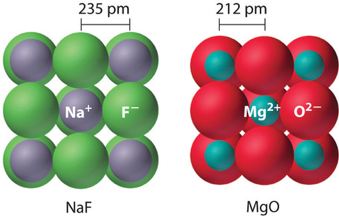• Lattice energy is dependent on two main factors:
• Radius of ions. Larger the ions, lower the lattice energy. For example, KCl has lower lattice energy than NaCl because potassium ion is larger than sodium ion in terms of ionic radius.
• Charge of ions. Greater the charge, higher the lattice energy. For example, MgCl2 has greater lattice energy than NaCl because magnesium ion has a +2 charge while sodium ion has a +1 charge.

Steps of Dissolution

• Dissolution of ionic compounds occurs in three steps:
1. Separation of solvent molecules is endothermic as energy is absorbed to overcome intermolecular forces present between molecules. Hydrogen bonds, dipole-dipole forces and dispersion forces are present between water molecules. Each water molecule can form up to four hydrogen bonds, causing it to have a relatively high boiling point for a molecule of its size.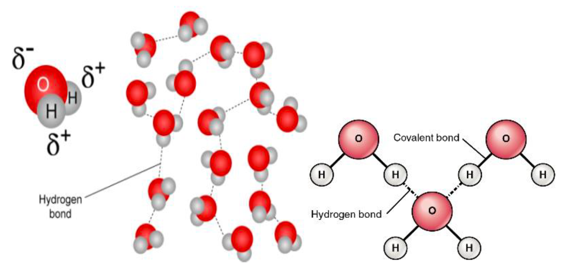Figure: covalent bonds are intramolecular bonds which are much stronger than intermolecular hydrogen bonds between water molecules.

1. Dissociation of solutes (ions) is endothermic as energy is absorbed to overcome the electrostatic forces present between ions in the lattice structure of the ionic compound.
1. Hydration of ions: dissociated ions are surrounded by separated solvent molecules. Intermolecular forces are formed between solutes and solvents, releasing energy in the process. Hydration is an exothermic process.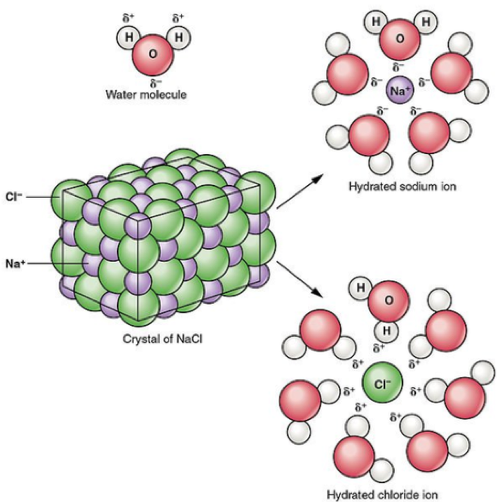Figure: Dissociation occurs as ionic bonds in NaCl are broken. Energy absorbed must exceed the ionic compound’s lattice energy. This is followed by the hydration of Na+ and Cl-. Hydration involves formation of ion-dipole forces which release energy. When NaCl dissolves in water, Na+ ions are attracted to oxygen atoms of water molecules as they are partially negative. Cl- ions are attracted to partially positive hydrogen atoms of water.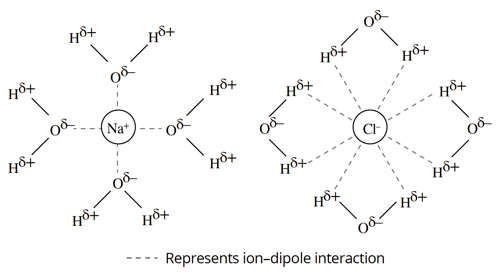Figure: A simple drawing of the hydration process of dissociated Na+ and Cl- ions. Each ion is hydrated (surrounded) by exactly four water molecules.   Chlorine ions are usually hydrated by more water molecules than sodium ions because of their larger ionic radius. This is not demonstrated by the drawing.

Table: Changes in enthalpy and entropy of each stage of dissolution of ionic compounds.

 DeltaH DeltaS Step 1: Separating solvent molecules Positive; endothermic Positive Step 2: Solute dissociation Positive: endothermic Positive Step 3: Ion hydration Negative: exothermic Negative

Factors Affecting Solubility

• The forces of attraction between the ions in the solid. Greater the attraction, lower the solubility as lattice energy is higher.
• The forces of attraction between the water molecules. Greater the attraction, lower the solubility as more energy is required to separate the solvent molecules.
• The forces of attraction between the particles of solid and solvent. Greater the attraction, higher the solubility as hydration of ions occurs more readily.

Change of Enthalpy During Dissolution

 ·       The overall change in enthalpy of dissolution of ionic compounds accounts for the change in enthalpy of each of the three steps of dissolution: separation of solvent molecules, dissociation of ionic lattice structure and hydration of free ions. ·       Dissolution is endothermic if the energy absorbed during solvent separation and dissociation is greater than what is released during ion hydration. An endothermic dissolution would decrease the temperature of the surrounding environment. Thus, the beaker in which the dissolution occurs will feel colder. ·       Dissolution is exothermic if the energy absorbed during solvent separation and dissociation is less than what is released during ion hydration. An exothermic dissolution would increase the temperature of the surrounding environment. Thus, the beaker in which the dissolution occurs will feel warmer.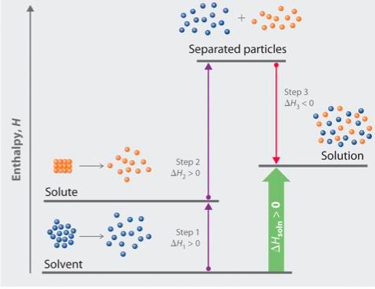Figure: Energy loss is less than what was absorbed in an endothermic reactionFigure: Energy loss is greater than what was absorbed in an exothermic reaction

﻿